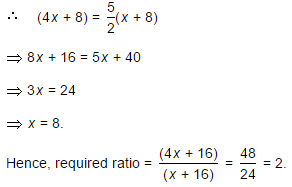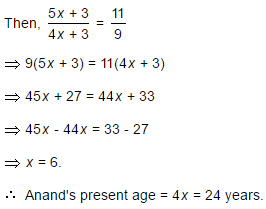Courses

# Test: Problems On Ages - 4

## 5 Questions MCQ Test General Aptitude for GATE 2020 | Test: Problems On Ages - 4

Description
This mock test of Test: Problems On Ages - 4 for Quant helps you for every Quant entrance exam. This contains 5 Multiple Choice Questions for Quant Test: Problems On Ages - 4 (mcq) to study with solutions a complete question bank. The solved questions answers in this Test: Problems On Ages - 4 quiz give you a good mix of easy questions and tough questions. Quant students definitely take this Test: Problems On Ages - 4 exercise for a better result in the exam. You can find other Test: Problems On Ages - 4 extra questions, long questions & short questions for Quant on EduRev as well by searching above.
QUESTION: 1

### Father is aged three times more than his son Ronit. After 8 years, he would be two and a half times of Ronit's age. After further 8 years, how many times would he be of Ronit's age?

Solution:

Let Ronit's present age be x years. Then, father's present age =(x + 3x) years = 4x years.QUESTION: 2

### The sum of ages of 5 children born at the intervals of 3 years each is 50 years. What is the age of the youngest child?

Solution:

Let the ages of children be x, (x + 3), (x + 6), (x + 9) and (x + 12) years.

Then, x + (x + 3) + (x + 6) + (x + 9) + (x + 12) = 505x = 20x = 4.Age of the youngest child = x = 4 years.

QUESTION: 3

### A father said to his son, "I was as old as you are at the present at the time of your birth". If the father's age is 38 years now, the son's age five years back was:

Solution:

Let the son's present age be x years. Then, (38 - x) = x2x = 38.x = 19.Son's age 5 years back (19 - 5) = 14 years.

QUESTION: 4

A is two years older than B who is twice as old as C. If the total of the ages of A, B and C be 27, the how old is B?

Solution:

Let C's age be x years. Then, B's age = 2x years. A's age = (2x + 2) years.(2x + 2) + 2x + x = 275x = 25x = 5.

Hence, B's age = 2x = 10 years.

QUESTION: 5

Present ages of Sameer and Anand are in the ratio of 5 : 4 respectively. Three years hence, the ratio of their ages will become 11 : 9 respectively. What is Anand's present age in years?

Solution:

Let the present ages of Sameer and Anand be 5x years and 4x years respectively.